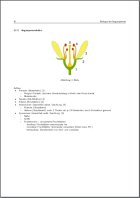# Korseby Online - LaTeX Templates

Here you can find some useful additions, functions and tricks for LaTeX.

### Some SamplesPicture & Keywords

If you want some features, you need some packages and extensions which come with LaTeX preinstalled. These are the packages which I am using:

 \documentclass[a4paper,10pt,twoside]{article} \usepackage[left=2cm,right=2cm,top=3cm,bottom=2cm,includeheadfoot]{geometry} \usepackage{textcomp} \usepackage{ngerman} \usepackage{eurosym} \usepackage[applemac]{inputenc} \usepackage{fancyhdr} \usepackage[pdftex]{graphicx}

### Definitions

Definitions are rather simple as you can see here:

 \def \TITLE{BIG Title} \def \SUBTITLE{Under Title} \def \AUTHOR{Kristian Peters}

Later you can access them via \SUBTITLE for example.

### Where's the sign for Degree?

If you want the degree sign you'll have to do something tricky. Simply user \Celsius in your text.

 \def \Celsius{\mathrm{^{\circ}C}}

### A simple way for a good looking math-mode?

I do not like the standard way mathematic formulas look like. So I redifed the math-mode a little. Simply use \M{formula}.

 \newcommand{\M}{$\mathrm{#1}$}

If you only want a little heading and some keywords which do not take so much space, you can make use of these:

 % Heading \newcommand{\HEADING}{\vspace{1pt}\paragraph*{#1}\ \\} % Keywords \newcommand{\SP}{\makebox[\textwidth][t]{\begin{tabular}{p{0.2cm} p{16cm}}\hfill$\mathrm{\bullet}$ & #1\end{tabular}}} \newcommand{\SSP}{\makebox[\textwidth][t]{\begin{tabular}{p{0.95cm} p{15.25cm}}\hfill$\mathrm{\circ}$ & #1\end{tabular}}} \newcommand{\SSSP}{\makebox[\textwidth][t]{\begin{tabular}{p{1.325cm} p{14.885cm}}\hfill$\mathrm{\cdot}$ & #1\end{tabular}}} % Enumeration \newcommand{\AP}{\makebox[\textwidth][t]{\begin{tabular}{p{0.2cm} p{16cm}}\hfill#1. & #2\end{tabular}}} \newcommand{\AAP}{\makebox[\textwidth][t]{\begin{tabular}{p{0.95cm} p{15.25cm}}\hfill#1. & #2\end{tabular}}} \newcommand{\AAAP}{\makebox[\textwidth][t]{\begin{tabular}{p{1.325cm} p{14.885cm}}\hfill#1. & #2\end{tabular}}}

You can access them as follows:

### Tricks with Pics

Inserting pictures is always a bit pedestrian, so I've written a little makro that automatically adds a figure text. There are also makros for 2 or 3 pictures at once. (Note: The lines at the html-code have linebreaks. For best achievements you better should use the samples from the whole template file.)

 % one picture \newcommand{\PIC}{\begin{figure}[!h]\begin{center}\includegraphics[width=#1]{#2} \caption{#3}\end{center}\end{figure}} % two pictures in a row \newcommand{\PPIC}{\begin{figure}[!h]\begin{minipage}{0.5\textwidth} \begin{center}\includegraphics[width=7.5cm]{#1}\caption{#2}\end{center} \end{minipage}\hfill\begin{minipage}{0.5\textwidth}\begin{center} \includegraphics[width=7.5cm]{#3}\caption{#4}\end{center}\end{minipage} \hfill\end{figure}} % three pictures in a row \newcommand{\PPPIC}{\begin{figure}[!h]\begin{minipage}{0.33\textwidth} \begin{center}\includegraphics[width=5cm]{#1}\caption{#2}\end{center} \end{minipage}\hfill\begin{minipage}{0.33\textwidth}\begin{center} \includegraphics[width=5cm]{#3}\caption{#4}\end{center}\end{minipage} \hfill\begin{minipage}{0.33\textwidth}\begin{center} \includegraphics[width=5cm]{#5}\caption{#6}\end{center}\end{minipage} \hfill\end{figure}}

And in the text, you can access them as follows:

 \vspace{7pt}\subsubsection{Bilder} \PIC{13cm}{data/datei.pdf}{einfaches Bild} \PPIC{data/Liriodendron_tulipifera.jpg}{zweifaches Bild 1} {data/Michaelia_champac_1.jpg}{zweifaches Bild 2} \PPPIC{data/Liriodendron_tulipifera.jpg}{dreifaches Bild 1} {data/Michaelia_champac_1.jpg}{dreifaches Bild 2} {data/Michaelia_champac_2.jpg}{dreifaches Bild 3}

There are also other things that are not so interesting like page numbering and so on... But you can download the whole template here.